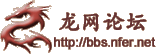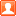##用户名 Email 自动登录 找回密码 密码 注册
 搜索

# [办公] EXCEL随机函数实例发表于 2010-6-18 09:16 | 显示全部楼层 |阅读模式
 excel中如何随机选择不重复的数： excel中带有一个随机函数rand（），但rand（）只选取0～1之间的随机数，而且两个数不保证不重复。因此就用到了函数rank。 1、假设要在1～100中随机抽取5个不重复的数。 2、在A1～A100中输入＝rand（）。 ；也就是在A1～A100中随机抽取了100个0～1之间的随机数。 3、在C1中输入=RANK(A1,A:A)；C2中输入=RANK(A2,A:A)；C3中输入=RANK(A3,A:A)……以此类推。 ；目标单元格C1～C5。 ；其实，C1~C5中显示的是A1～A5在100个随机数中的排序。 4、已成功抽取1～100中的5个随机数。按ctrl＋r刷新。 1、生成随机数字 (1)生成随机数比较简单，=rand()即可生成0-1之间的随机数； (2)如果要是整数，就用=int(rand())*10，表示0至9的整数，以此类推； (3)如果要生成a与b之间的随机实数，就用=rand()*(b-a)+a，如果是要整数就用=int(rand()*(b-a))+a；稍微扩充一下，就能产生固定位数的整数了。 注意：如果要使用函数rand()生成一随机数，并且使之不随单元格计算而改变，可以在编辑栏中输入“=rand()”，保持编辑状态，然后按F9，将公式永久性地改为随机数。不过，这样只能一个一个的永久性更改，如果数字比较多，也可以全部选择之后，另外选择一个合适的位置粘贴，粘贴的方法是点击右键，选择“选择性粘贴”，然后选择“数值”，即可将之前复制的随机数公式产生的数值（而不是公式）复制下来！ 2、产生随机字母 随机小写字母：=CHAR(INT(RAND()*26)+97) 随机大写字母：=CHAR(INT(RAND()*26)+65) 随机大小写混合字母：=CHAR(INT(RAND()*26)+if(INT(RAND()*2)=0,65,97)) 3、产生随机的六位数的字母和数字混合 =CONCATENATE(IF(INT(RAND()*2)=0,CHAR(INT(RAND()*9+48)),IF(INT(RAND()*2)=0,CHAR(INT(RAND()*25+65)),CHAR(INT(RAND()*25+97)))),IF(INT(RAND()*2)=0,CHAR(INT(RAND()*9+48)),IF(INT(RAND()*2)=0,CHAR(INT(RAND()*25+65)),CHAR(INT(RAND()*25+97)))),IF(INT(RAND()*2)=0,CHAR(INT(RAND()*9+48)),IF(INT(RAND()*2)=0,CHAR(INT(RAND()*25+65)),CHAR(INT(RAND()*25+97)))),IF(INT(RAND()*2)=0,CHAR(INT(RAND()*9+48)),IF(INT(RAND()*2)=0,CHAR(INT(RAND()*25+65)),CHAR(INT(RAND()*25+97)))),IF(INT(RAND()*2)=0,CHAR(INT(RAND()*9+48)),IF(INT(RAND()*2)=0,CHAR(INT(RAND()*25+65)),CHAR(INT(RAND()*25+97)))),IF(INT(RAND()*2)=0,CHAR(INT(RAND()*9+48)),IF(INT(RAND()*2)=0,CHAR(INT(RAND()*25+65)),CHAR(INT(RAND()*25+97))))) 4、随机不重复数字序列的生成方法 有些情形下，我们需要生成一个不重复的随机序列。 比如：我们要模拟洗牌，将一副扑克牌去掉大小怪后剩下的52张打乱。 比较笨的方法是在1-52间每生成一个随机数后，检查该随机数是否出现过，如果是第一次出现，就放到序列里，否则重新生成一个随机数作检查。在 excel worksheet里面用这种办法，会造成if多层嵌套，不胜其烦，在VBA里面做简单一些，但是效率太差，越到序列的后端，效率越差。 当然也有比较好的办法，在VBA里面，将a(1)-a(52)分别赋予1-52，然后做52次循环，例如，第s次生成一个1-52间的随机数r，将a(s)与a(r)互换，这样的话，就打乱了原有序列，得到一个不重复的随机序列。 VBA里这个算法是很容易实现的，但是，出于通用性和安全考虑，有的时候我们并不希望用VBA，我们来看看在worksheet里面如何利用内置函数实现这个功能。 (1)在A1-A52间填入"=INT(RAND()*52)+1"，产生1-52间的随机数，注意这里是有重复的 (2)在B1-B52间填入1-52 (3)在C54-BB54填入1-52 (4)在C1填入"=IF(ROW()=C\$54,INDEX(B\$1:B\$52,INDEX(\$A\$1:\$A\$52,C\$54)),IF(ROW()=INDEX(\$A\$1:\$A\$52,C\$54),INDEX(B\$1:B\$52,C\$54),B1))"。 分项解释： a:ROW()=C\$54，如果当前行等于当前交换所排的序号 b:INDEX(B\$1:B\$52,INDEX(\$A\$1:\$A\$52,C\$54))，返回在B1到B52中选择A1:A52中的第C54个值 c:IF(ROW()=INDEX(\$A\$1:\$A\$52,C\$54)，否则的话，如果当前行等于A1:A52中第C54个值，则： d:INDEX(B\$1:B\$52,C\$54)，返回B1:B52中的第C54个值 e:若以上条件都不满足，则返回B1 (5)将C1复制到C1:BA52这个区域里面 (6)在BA1:BA52中，我们就得到了一个不重复的随机序列，按F9可以生成一个新序列。 随机产生六位数字密码=INT(RAND()*(899999-10001))+100001 EXCEL生成前2位是大写字母，中间4位是小写字母，后两位是数字 =CHAR(65+INT(RAND()*16))&CHAR(65+INT(RAND()*16))&CHAR(97+INT(RAND()*16))&CHAR(97+INT(RAND()*16))&CHAR(97+INT(RAND()*16))&CHAR(97+INT(RAND()*16))&INT(RAND()*10)&INT(RAND()*10)
 您需要登录后才可以回帖 登录 | 注册 本版积分规则 回帖后跳转到最后一页

GMT+8, 2022-9-26 17:48